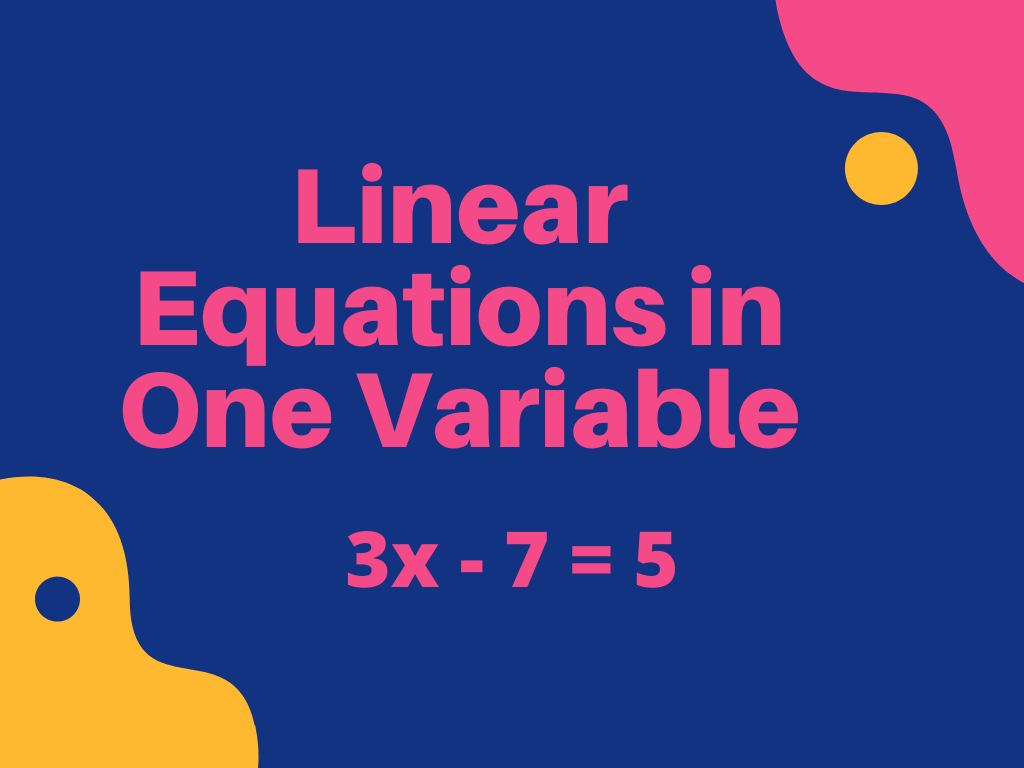## Linear Equations in One Variable Class 8th

Introduction We are familiar with algebraic expressions and equations because we have already studied these in the previous class. Algebraic expressions are the mathematical expressions that have the combination of numbers and the English alphabet letters. In the equations, there is the equality sign (=) that is not used in algebraic expressions. Some examples of …